### Localizations of a ring at maximal ideals correspond to stalks of a sheaf

We have previously observed that localizing a finitely generated algebra at a specific element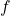yields a local ring which is exactly the set of regular functions on the distinguished open set, or in other words, the sections of the sheaf of regular functions on. That is to sayーwe have the concept of a (pre)sheaf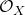, which ‘collects together’ regular functions that are defined on the open sets of a topological space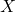, and we have shown that if we look at the functions that are assigned to special types of open sets (distinguished open sets), they correspond to the localizations of a finitely generated algebra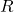at specific elements of that algebra. (Notably, this means they have global representations as polynomial quotients over each.) However, what happens if we want to look at the behavior of these functionsーsections of the sheafーnear a specific point in the topological space?

Say that the underlying topological space is. Given a specific point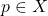, can we consider, instead, all of the functions in the sheaf which are ‘locally defined’ around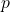? This is the stalk of the sheafat, and we will see that this corresponds exactly to the localization of the algebrato the maximal ideal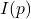.

Let’s motivate the problem a little more. The pointis itself a closed set. Take an arbitrary closed set inand imagine for a moment that its complement inwere given by distinguished open set; in such a case, we would identify the regular functions near this closed set, in particular on the entire surrounding distinguished open set, with fractions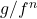,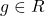, where by construction we know thatis nonzero on all of. We show this by looking at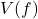and translating to an algebraic setting, showing that it corresponds to an ideal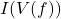which is a radical ideal generated by finitely many elements.

However,is of course not necessarily given by any straightforwardfor a single element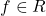! But recall that all pointscorrespond to maximal ideals in, and these maximal ideals form a natural denominator for our quotients.

Let’s propose the following: Take the maximal ideal incorresponding to. Call this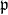. Previously, we localized to; in other words, we took quotients of ring elements where the denominators were guaranteed to be nonzero on our open set of interest. We can do something a little different here, and instead localize the ringto the complement of, so that we are taking quotients with all kinds of functions which are nonzero at. This is generally referred to as the localization ofto a prime ideal, or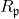.

Do the elements ofcorrespond to the regular functions defined on a neighborhood of? Let’s propose a natural homomorphism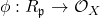that maps a quotient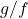,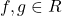with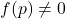, to the regular function given byon the distinguished open setwhich is, by definition, a neighborhood of(becausedoes not vanish at).

Recall that every regular function in a sufficiently small neighborhood of a point can be given as a quotient of polynomials where the denominator does not vanish at that point; therefore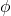is surjective. It is also easy to see thatis injective from the observation that distinguished open sets are the ‘smallest open sets’ of the Zariski topology. And there we have it: a mapping between stalks of the sheaf of regular functions at specific points and ring localizations at the maximal (and prime) ideals corresponding to those exact points!

February 11th, 2023 | Posted in Math